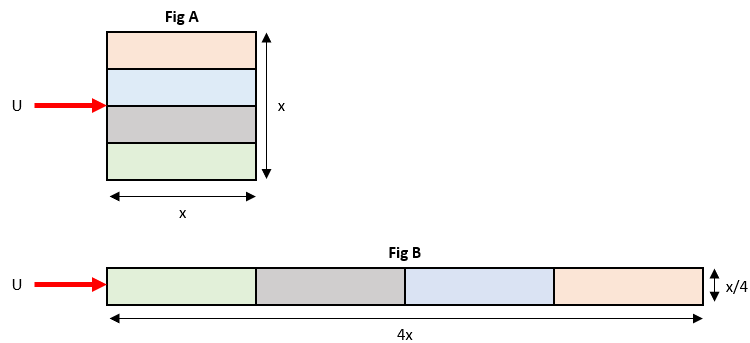## Optimizing for Drag

The square flat plate shown in Figure A is cut into four equal-sized pieces and arranged as shown in Figure B. Determine the ratio of the drag on the original plate (case A) to the drag on the plates shown in case B. Assume laminar boundary flow. Explain your answer physically.Hint
Drag force:
$$D_{f}=\frac{1}{2}\rho U^{2}C_{Df}A$$$where $$\rho$$ is density, $$U$$ is the speed of the object relative to the fluid, $$C_{Df}$$ is the drag coefficient, and $$A$$ is the cross sectional area. Hint 2 Drag coefficient: $$C_{Df}=\frac{1.328}{\sqrt{Re}}$$$
where $$Re$$ is the Reynold’s number.
Drag force:
$$D_{f}=\frac{1}{2}\rho U^{2}C_{Df}A$$$where $$\rho$$ is density, $$U$$ is the speed of the object relative to the fluid, $$C_{Df}$$ is the drag coefficient, and $$A$$ is the cross sectional area. For flat plates placed in parallel with flow, the drag coefficient is: $$C_{Df}=\frac{1.328}{\sqrt{Re}}$$$
where $$Re$$ is the Reynold’s number. Remember,
$$Re=\frac{Ul}{\nu}$$$where $$U$$ is the velocity, $$l$$ is the length, and $$\nu$$ is the kinematic viscosity. For case A: $$D_{fa}=\frac{1}{2}\rho U^{2}C_{Df}A$$ where $$A=x^2$$ and $$C_{Df}=\frac{1.328}{\sqrt{Re}}=\frac{1.328}{\sqrt{Ul/\nu}}=\frac{1.328}{\sqrt{Ux/\nu}}$$$
Thus,
$$D_{fa}=\frac{1}{2}\rho U^{2}\frac{1.328\sqrt{\nu}}{\sqrt{Ux}}x^2=0.644\rho U^{3/2}\cdot \sqrt{\nu}\cdot x^{3/2}$$$For case B: $$D_{fb}=\frac{1}{2}\rho U^{2}C_{Df}A$$ where $$A=(4x)(\frac{x}{4})=x^2$$ and $$C_{Df}=\frac{1.328}{\sqrt{Re}}=\frac{1.328}{\sqrt{Ul/\nu}}=\frac{1.328}{\sqrt{U4x/\nu}}$$$
Thus,
$$D_{fb}=\frac{1}{2}\rho U^{2}\frac{1.328\sqrt{\nu}}{\sqrt{4Ux}}x^2=\frac{1}{2}(0.644\rho U^{3/2}\cdot \sqrt{\nu}\cdot x^{3/2})$$\$
By comparing the two equations, the Drag force in case A is twice as much as case B. In case B, the boundary layer on the rear plate is thicker than on the front plate, meaning the shear stress is less on the rear plate than it is in configuration A. This gives less drag for case B, despite the total areas being equivalent.
The Drag force in case A is twice as much as case B. In case B, the boundary layer on the rear plate is thicker than on the front plate, meaning the shear stress is less on the rear plate than it is in configuration A. This gives less drag for case B, despite the total areas being equivalent.